# Deep Learning from Scratch and Using Tensorflow in PythonIn this article, we will learn how deep learning works and get familiar with its terminology — such as backpropagation and batch size

Originally published by Milad Toutounchian at https://towardsdatascience.com Deep learning is one of the most popular models currently being used in real-world, Data Science applications. It’s been an effective model in areas that range from image to text to voice/music. With the increase in its use, the ability to quickly and scalably implement deep learning becomes paramount. The rise of deep learning platforms such as Tensorflow, help developers implement what they need to in easier ways.

In this article, we will learn how deep learning works and get familiar with its terminology — such as backpropagation and batch size. We will implement a simple deep learning model — from theory to scratch implementation — for a predefined input and output in Python, and then do the same using deep learning platforms such as Keras and Tensorflow. We have written this simple deep learning model using Keras and Tensorflow version 1.x and version 2.0 with three different levels of complexity and ease of coding.

## Deep Learning Implementation from Scratch

Consider a simple multi-layer-perceptron with four input neurons, one hidden layer with three neurons and an output layer with one neuron. We have three data-samples for the input denoted as X, and three data-samples for the desired output denoted as yt. So, each input data-sample has four features.

``````# Inputs and outputs of the neural net:
import numpy as np

X=np.array([[1.0, 0.0, 1.0, 0.0],[1.0, 0.0, 1.0, 1.0],[0.0, 1.0, 0.0, 1.0]])
yt=np.array([[1.0],[1.0],[0.0]])
``````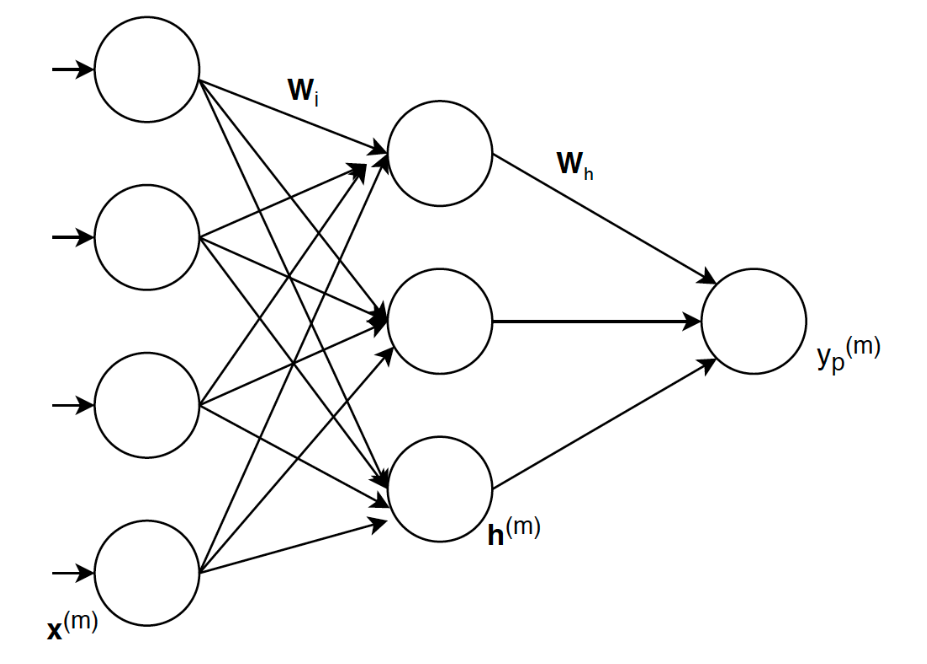The x*(m) in this figure is one-sample of Xh(m) is the output of the hidden layer for input x*(m), and W*i and W*h are the weights.

The goal of a neural net (NN) is to obtain weights and biases such that for a given input, the NN provides the desired output. But, we do not know the appropriate weights and biases in advance, so we update the weights and biases such that the error between the output of NN, yp(m), and desired ones, yt(m), is minimized. This iterative minimization process is called the NN training.

Assume the activation functions for both hidden and output layers are sigmoid functions. Therefore,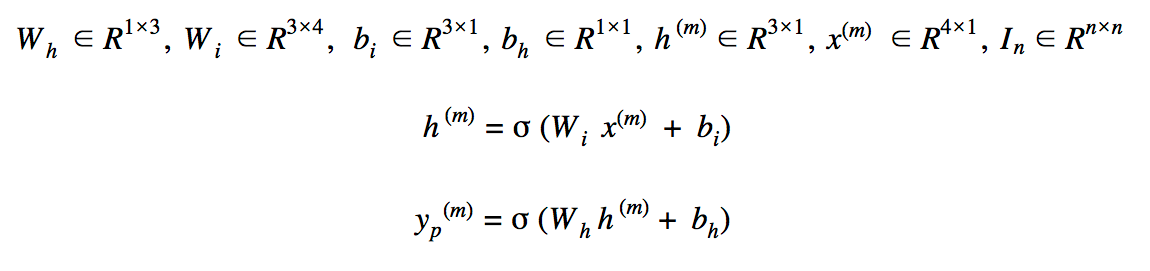The size of weights, biases and the relationships between input and outputs of the neural net

Where activation function is the sigmoid, m is the mth data-sample and yp(m) is the NN output.

The error function, which measures the difference between the output of NN with the desired one, can be expressed mathematically as: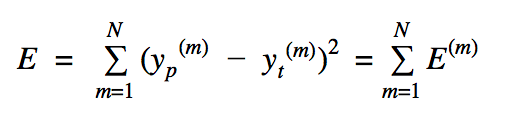The Error defined for the neural net which is squared error

The pseudocode for the above NN has been summarized below: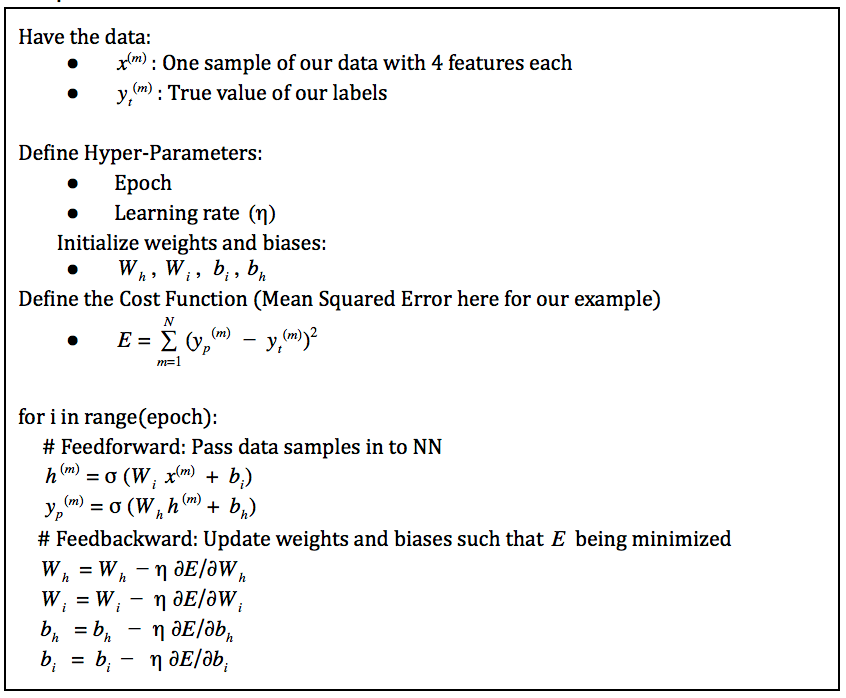pseudocode for the neural net training

From our pseudocode, we realize that the partial derivative of Error (E) with respect to parameters (weights and biases) should be computed. Using the chain rule from calculus we can write: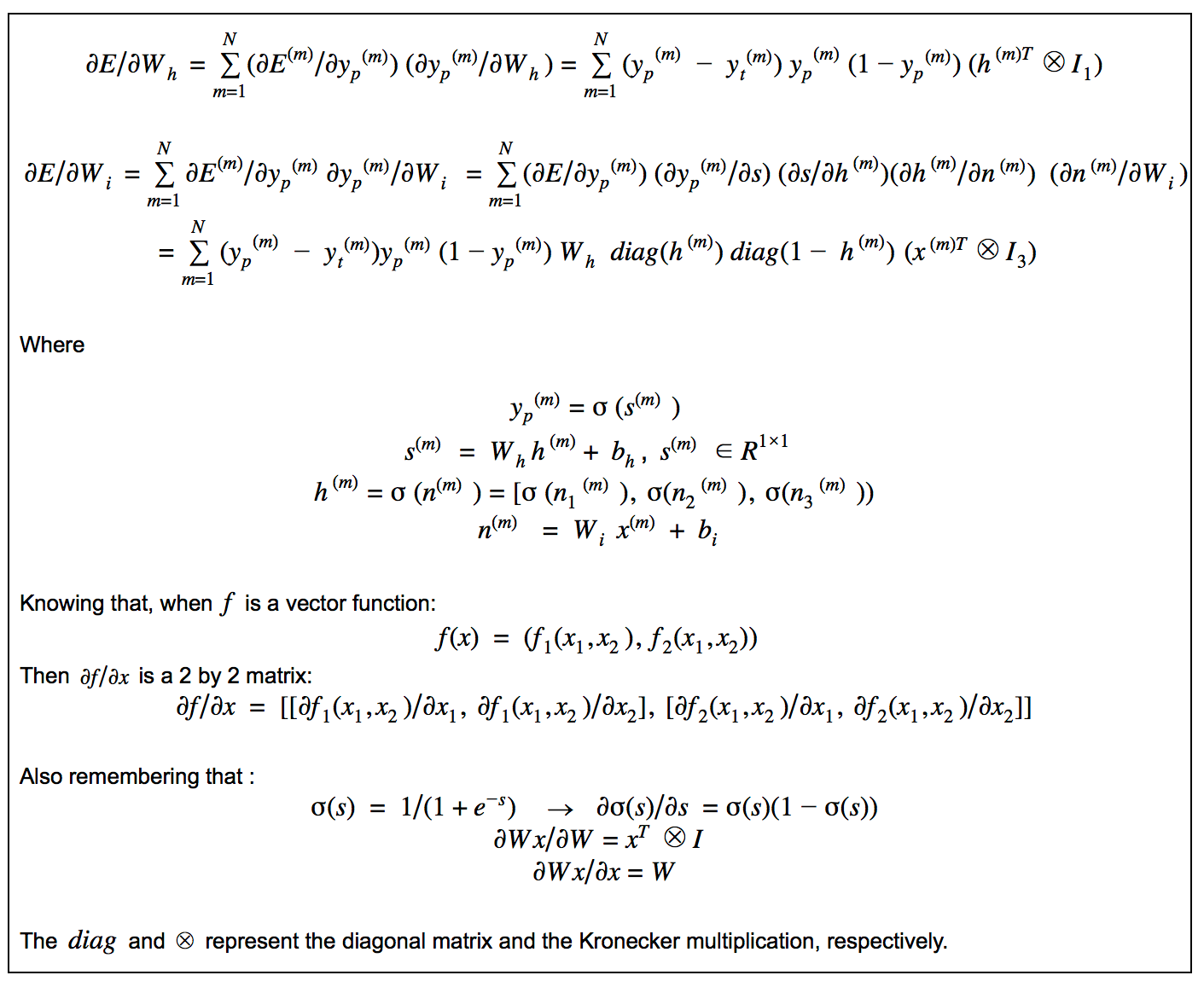We have two options here for updating the weights and biases in backward path (backward path means updating weights and biases such that error is minimized):

1. Use all *N * samples of the training data
2. Use one sample (or a couple of samples)

For the first one, we say the batch size is N. For the second one, we say batch size is 1, if use one sample to updates the parameters. So batch size means how many data samples are being used for updating the weights and biases.

You can find the implementation of the above neural net, in which the gradient of the error with respect to parameters is calculated Symbolically, with different batch sizes here.

As you can see with the above example, creating a simple deep learning model from scratch involves methods that are very complex. In the next section, we will see how deep learning frameworks can assist in introducing scalability and greater ease of implementation to our model.

## Deep Learning implementation using Keras, Tensorflow 1.x and 2.0

In the previous section, we computed the gradient of Error w.r.t. parameters from using the chain rule. We saw first-hand that it is not an easy or scalable approach. Also, keep in mind that we evaluate the partial derivatives at each iteration, and as a result, the Symbolic Gradient is not needed although its value is important. This is where deep-learning frameworks such as Keras and Tensorflow can play their role. The deep-learning frameworks use an AutoDiff method for numerical calculations of partial gradients. If you’re not familiar with AutoDiff, StackExchange has a great example to walk through.

The AutoDiff decomposes the complex expression into a set of primitive ones, i.e. expressions consisting of at most a single function call. As the differentiation rules for each separate expression are already known, the final results can be computed in an efficient way.

We have implemented the NN model with three different levels in Keras, Tensorflow 1.x and Tensorflow 2.0:

1- High-Level (Keras and Tensorflow 2.0): High-Level Tensorflow 2.0 with Batch Size 1

3- Low-Level (Tensorflow 1.x): Low-Level Tensorflow 1.x with Batch Size N

Code Snippets:

For the High-Level, we have accomplished the implementation using Keras and Tensorflow v 2.0 with model.train_on_batch:

``````# High-Level implementation of the neural net in Tensorflow:
model.compile(loss=mse, optimizer=optimizer)
for _ in range(2000):
for step, (x, y) in enumerate(zip(X_data, y_data)):
model.train_on_batch(np.array([x]), np.array([y]))
``````

In the Medium-Level using Tensorflow 1.x, we have defined:

``````E = tf.reduce_sum(tf.pow(ypred - Y, 2))
optimizer = tf.train.GradientDescentOptimizer(0.1)
grads = optimizer.compute_gradients(E, [W_h, b_h, W_o, b_o])
updates = optimizer.apply_gradients(grads)
``````

This ensures that in the for loop, the updates variable will be updated. For Medium-Level, the gradients and their updates are defined outside the for_loop and inside the for_loop updates is iteratively updated. In the Medium-Level using Tensorflow v 2.x, we have used:

``````# Medium-Level implementation of the neural net in Tensorflow

# In for_loop
with tf.GradientTape() as tape:
x = tf.convert_to_tensor(np.array([x]), dtype=tf.float64)
y = tf.convert_to_tensor(np.array([y]), dtype=tf.float64)
ypred = model(x)
loss = mse(y, ypred)
gradients = tape.gradient(loss, model.trainable_weights)
optimizer.apply_gradients(zip(gradients, model.trainable_weights))
``````

In Low-Level implementation, each weight and bias is updated separately. In the Low-Level using Tensorflow v 1.x, we have defined:

``````# Low-Level implementation of the neural net in Tensorflow:
E = tf.reduce_sum(tf.pow(ypred - Y, 2))
dE_dW_h = tf.gradients(E, [W_h])
dE_db_h = tf.gradients(E, [b_h])
dE_dW_o = tf.gradients(E, [W_o])
dE_db_o = tf.gradients(E, [b_o])
# In for_loop:
evaluated_dE_dW_h = sess.run(dE_dW_h,
feed_dict={W_h: W_h_i, b_h: b_h_i, W_o: W_o_i, b_o: b_o_i, X: X_data.T, Y: y_data.T})
W_h_i = W_h_i - 0.1 * evaluated_dE_dW_h
evaluated_dE_db_h = sess.run(dE_db_h,
feed_dict={W_h: W_h_i, b_h: b_h_i, W_o: W_o_i, b_o: b_o_i, X: X_data.T, Y: y_data.T})
b_h_i = b_h_i - 0.1 * evaluated_dE_db_h
evaluated_dE_dW_o = sess.run(dE_dW_o,
feed_dict={W_h: W_h_i, b_h: b_h_i, W_o: W_o_i, b_o: b_o_i, X: X_data.T, Y: y_data.T})
W_o_i = W_o_i - 0.1 * evaluated_dE_dW_o
evaluated_dE_db_o = sess.run(dE_db_o,
feed_dict={W_h: W_h_i, b_h: b_h_i, W_o: W_o_i, b_o: b_o_i, X: X_data.T, Y: y_data.T})
b_o_i = b_o_i - 0.1 * evaluated_dE_db_o
``````

As you can see with the above low level implementation, the developer has more control over every single step of numerical operations and calculations.

## Conclusion

We have now shown that implementing from scratch even a simple deep learning model by using Symbolic gradient computation for weight and bias updates is not an easy or scalable approach. Using deep learning frameworks accelerates this process as a result of using AutoDiff, which is basically a stable numerical gradient computation for updating weights and biases.

Thanks for reading

If you liked this post, share it with all of your programming buddies!

Follow us on Facebook | Twitter

## PyTorch for Deep Learning | Data Science | Machine Learning | Python

PyTorch for Deep Learning | Data Science | Machine Learning | Python. PyTorch is a library in Python which provides tools to build deep learning models. What python does for programming PyTorch does for deep learning. Python is a very flexible language for programming and just like python, the PyTorch library provides flexible tools for deep learning.

## Data Science With Python Training | Python Data Science Course | Intellipaat

🔵 Intellipaat Data Science with Python course: https://intellipaat.com/python-for-data-science-training/In this Data Science With Python Training video, you...

## PyTorch for Deep Learning | Data Science | Machine Learning | Python

PyTorch is a library in Python which provides tools to build deep learning models. What python does for programming PyTorch does for deep learning.

## 6 Best Python IDEs for Data Science & Machine Learning 

6 Best Python IDEs for Data Science & Machine Learning  - An IDE (Integrated Development Environment) is used for software development. An IDE may have a compiler, debugger, and all the other requirements needed for software development. IDEs help in consolidating different aspects of a computer program

## Data Science Projects | Data Science | Machine Learning | Python

Practice your skills in Data Science with Python, by learning and then trying all these hands-on, interactive projects, that I have posted for you.